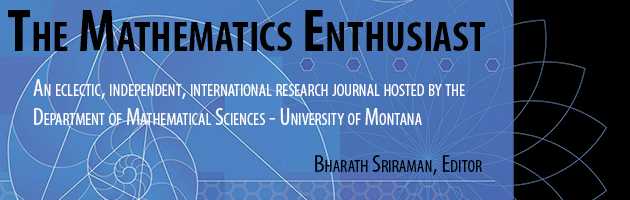•
•15

3

#### Abstract

The notion of function is considered one of the most difficult parts of the common lower secondary curriculum. In this paper we discuss the potential role played by linear functions, invariably used as first examples of this new notion. As empirical basis we use a praxeological analysis of the function chapter in four Indonesian lower secondary textbooks. The main point of our analysis is that the class of functions of type 𝑓(𝑥)=𝑎𝑥 (where a is some given number) does not appear explicitly at the level of theory, neither in the sense of being given a name, or in the sense that properties of the class is studied. We discuss the implications of this for students’ learning of the more general (theoretical) notion of function.

467

482

COinS